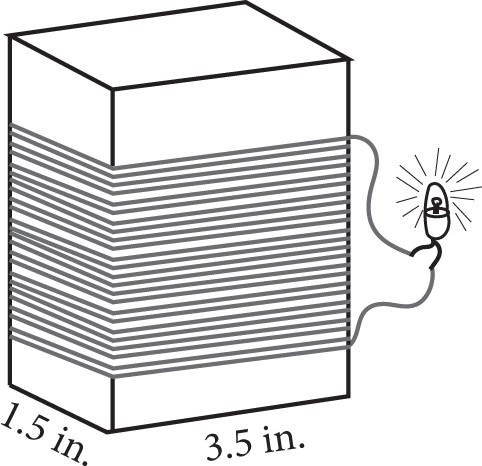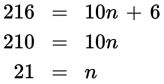# SAT Math Multiple Choice Question 381: Answer and Explanation

### Test Information

Question: 381

6.Geraldine is making a simple AC electric generator for a science project using copper wire, cardboard, a nail, and magnets. The first step in building the generator is wrapping the wire around and around a rectangular prism made from the cardboard and connecting it to a small lightbulb, as shown in the figure. If Geraldine has 18 feet of wire and needs to leave 3 inches on each end to connect to the lightbulb, how many times can she wrap the wire around the cardboard prism?

• A. 21
• B. 28
• C. 35
• D. 42

Explanation:

A

Difficulty: Medium

Category: Heart of Algebra / Linear Equations

Strategic Advice: Sometimes writing an equation is the quickest route to answering a question. Assign a variable to the unknown, write the equation in words, and then translate from English into math.

Getting to the Answer: The unknown in this question is the number of times Geraldine can wrap the wire around the prism. Call this n. Now, write an equation in words: Total amount of wire equals distance around the prism times the number of wraps plus the extra on the ends. To fill in the numbers, you'll need to make a few calculations. Because the dimensions of the prism are given in inches, convert the amount of wire to inches as well: 18 ft = 18 × 12 = 216 inches. Next, figure out the distance around the prism using the picture. Don't forget, you have to go all the way around: 1.5 + 3.5 + 1.5 + 3.5 = 10 inches. Finally, read the question again to determine that the extra on the ends is 3 + 3 = 6 inches. Now you're ready to translate from English into math to write your equation, and then solve it.Geraldine can wrap the wire around the prism 21 times.